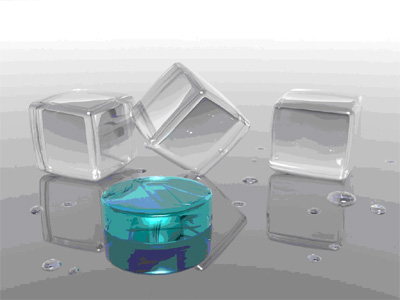COURSE DESCRIPTIONUnderstanding the following: Points and Lines. The Language and Logic of Geometry. From Reflections to Congruence. Proofs using Congruence. Polygons and Symmetry. Perimeters and Areas. Three-Dimensional Figures. Surface Areas and Volumes.

CHAPTERSCh1. – Nets and Drawings
Ch2. – Measuring Segments
Ch3. – Exploring Angle Pairs
Ch4. – Midpoint and Distance
Ch5. – Patterns and Inductive Resoning
Ch6. – Biconditionals and Definitions
Ch7. – Reasoning in Algebra and Geometry
Ch8. – Lines and Angles
Ch9. – Proving Lines Parallel
Ch10. – Parallel Lines and Triangles
Ch11. – Equations of Lines
Ch12. – Congruent Figures
Ch13. – Triangle Congruence
Ch14. – Corresponding Parts of Congruent Triangles
Ch15. – Congruence in Right Triangles
Ch16. – Midsegments of Triangles
Ch17. – Bisectors in Triangles
Ch18. – Course Survey
Ch19. – Indirect Proof
Ch20. – The Polygon Angle-Sum Theorems
Ch22. – Conditions
Ch23. – Polygons in the Coordinate Plane
Ch24. – Ratios and Proportions
Ch25. – Proving Triangles Similar
Ch26. – The Pythagorean Theorem
Ch27. – Trigonometry
Ch28. – Translations
Ch29. – Rotations
Ch30. – Dilations
Ch31. – Area of Parallelograms
Ch32. – Areas of Regular Polygons
Ch33. – Trigonometry and Area
Ch34. – Areas of Circles and Sectors
Ch35. – Space Figures and Cross Sections
Ch36. – Surface Areas of Pyramids and Cones
Ch37. – Volumes of Pyramids and Cones